Скачать презентацию Automated Reasoning Matt Whipple and Brian Vees

• Количество слайдов: 20Automated Reasoning Matt Whipple and Brian VeesOverview What is automated reasoning? n Properties of inference procedures n Theorem prover n Diagnosis with first principles n Logic circuit design and validation n Program verification and validation n Conclusion n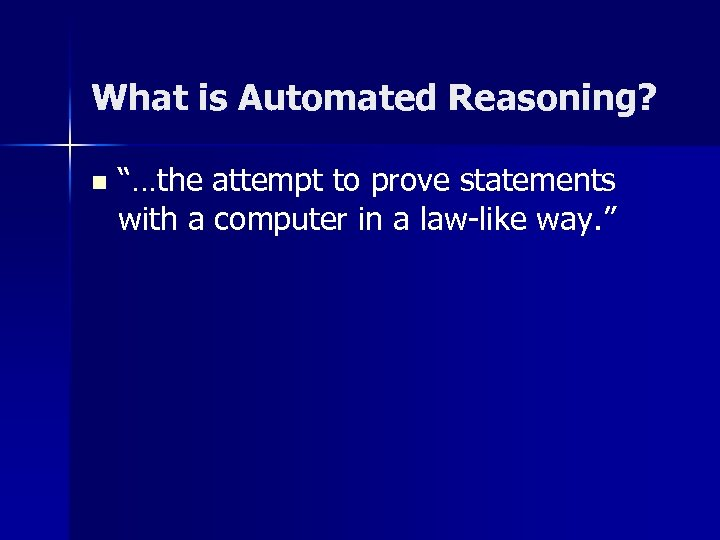What is Automated Reasoning? n “…the attempt to prove statements with a computer in a law-like way. ”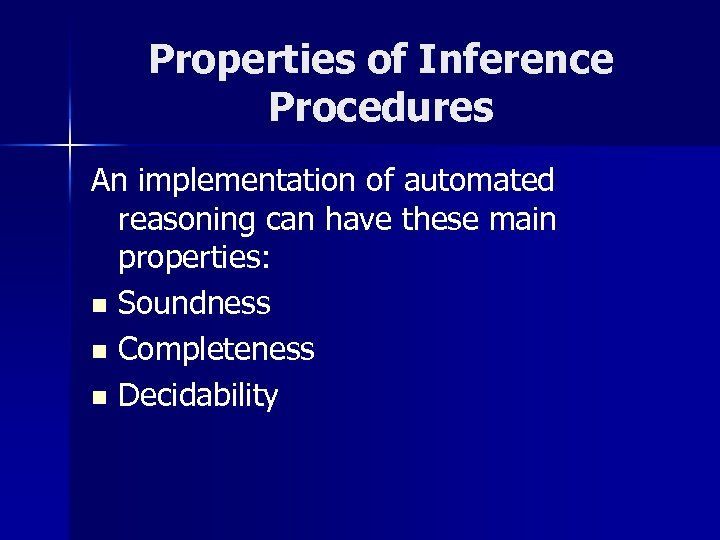Properties of Inference Procedures An implementation of automated reasoning can have these main properties: n Soundness n Completeness n Decidability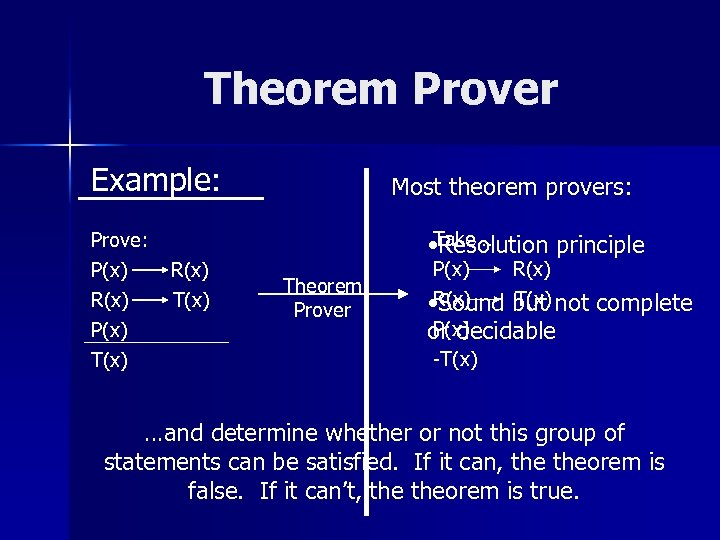Theorem Prover Example: Prove: P(x) R(x) P(x) T(x) R(x) T(x) Most theorem provers: Take… • Resolution principle Theorem Prover P(x) R(x) T(x) • Sound but not complete P(x) or decidable -T(x) …and determine whether or not this group of statements can be satisfied. If it can, theorem is false. If it can’t, theorem is true.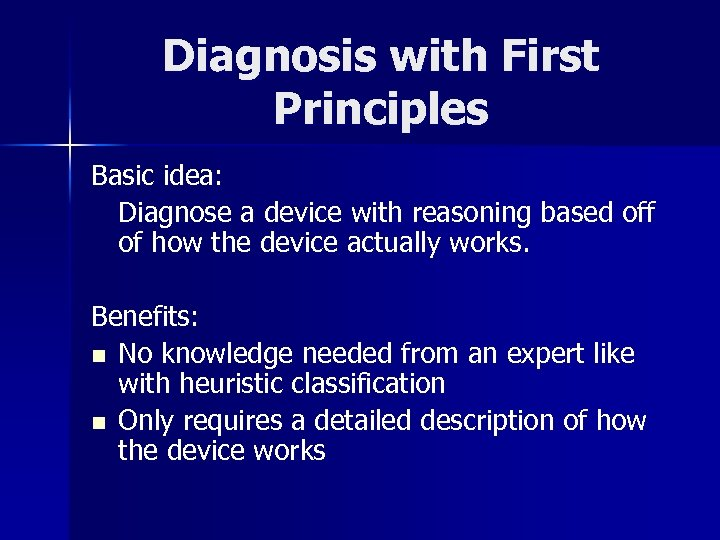Diagnosis with First Principles Basic idea: Diagnose a device with reasoning based off of how the device actually works. Benefits: n No knowledge needed from an expert like with heuristic classification n Only requires a detailed description of how the device works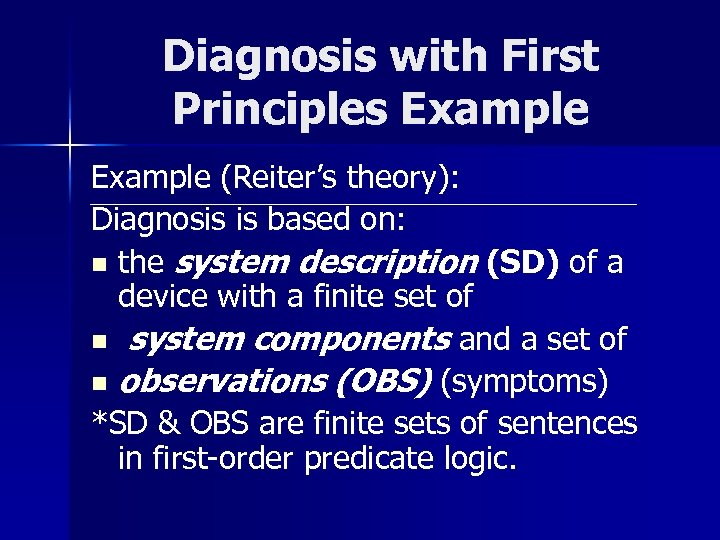Diagnosis with First Principles Example (Reiter’s theory): Diagnosis is based on: n the system description (SD) of a device with a finite set of n system components and a set of n observations (OBS) (symptoms) *SD & OBS are finite sets of sentences in first-order predicate logic.Diagnosis with First Principles Example (Reiter’s theory): A diagnosis for the set ( SD, COMPONENTS, OBS ) is a set of faulty components. A component is part of this faulty set only if assuming it is non-faulty creates a contradiction with the device description and its symptoms. The idea - form a set that consists of members of each faulty set.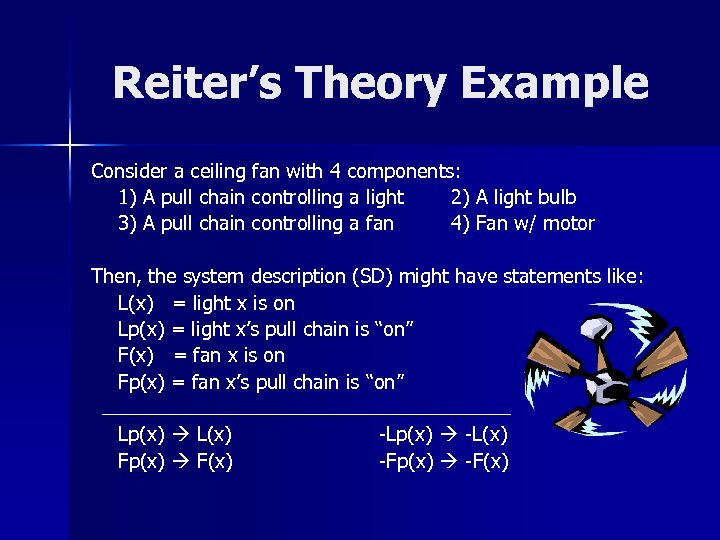Reiter’s Theory Example Consider a ceiling fan with 4 components: 1) A pull chain controlling a light 2) A light bulb 3) A pull chain controlling a fan 4) Fan w/ motor Then, the system description (SD) might have statements like: L(x) = light x is on Lp(x) = light x’s pull chain is “on” F(x) = fan x is on Fp(x) = fan x’s pull chain is “on” Lp(x) L(x) Fp(x) F(x) -Lp(x) -L(x) -Fp(x) -F(x)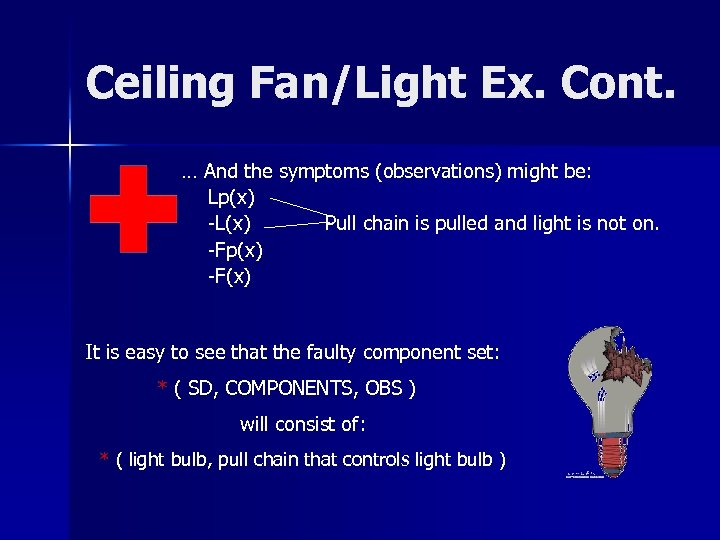Ceiling Fan/Light Ex. Cont. … And the symptoms (observations) might be: Lp(x) -L(x) Pull chain is pulled and light is not on. -Fp(x) -F(x) It is easy to see that the faulty component set: * ( SD, COMPONENTS, OBS ) will consist of: * ( light bulb, pull chain that controls light bulb )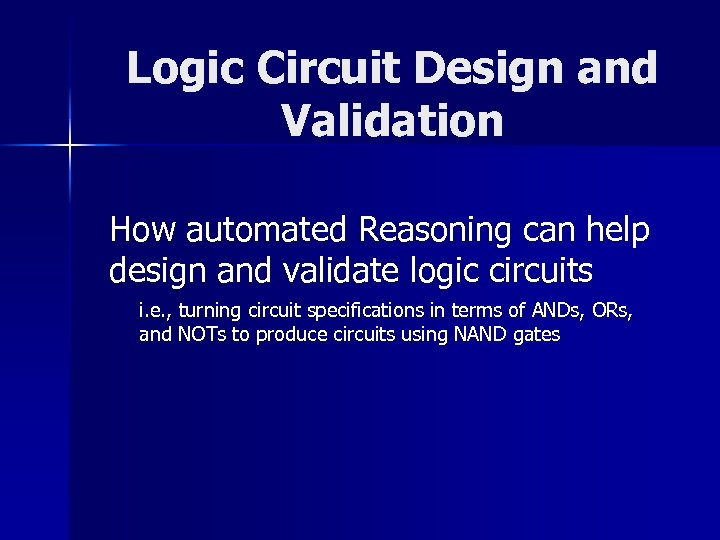Logic Circuit Design and Validation How automated Reasoning can help design and validate logic circuits i. e. , turning circuit specifications in terms of ANDs, ORs, and NOTs to produce circuits using NAND gatesCircuit Design Example Converting from various gates to purely NAND o 1 = or(and(i 1, i 2), not (i 3)) o 2 = and(not(i 3), i 2) not(x) g nand(x, x) or( x, y) g nand(not( x), not(y)) and(x, y) g not(nand(x, y)) Simplified… nand(x, x), nand(x, x)) g x. DemodulationDemodulation The substitutuing or rewriting of one term by an equivalent one n Applied when the first clause unifies with the term we are attempting to rewrite n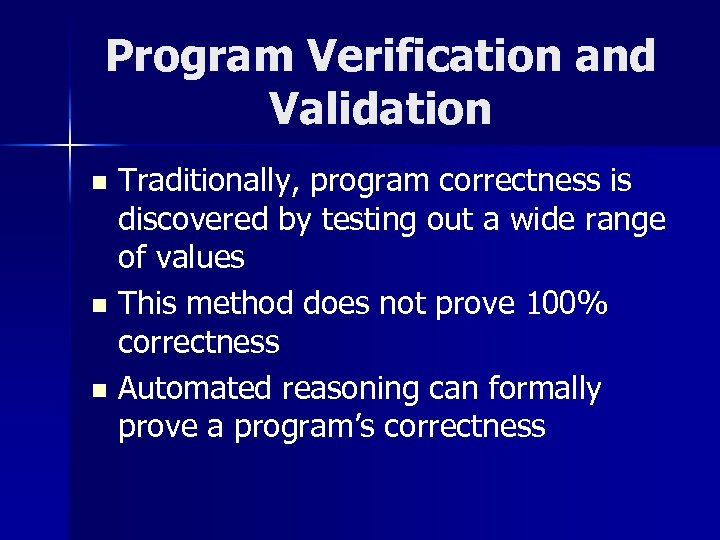Program Verification and Validation Traditionally, program correctness is discovered by testing out a wide range of values n This method does not prove 100% correctness n Automated reasoning can formally prove a program’s correctness n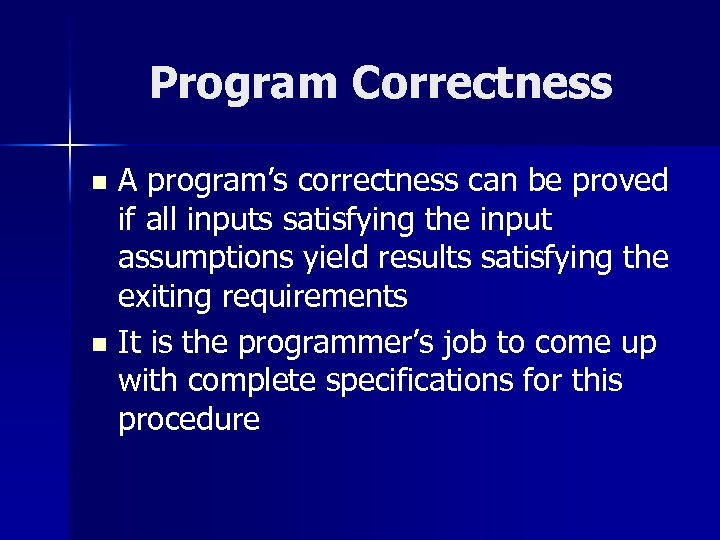Program Correctness A program’s correctness can be proved if all inputs satisfying the input assumptions yield results satisfying the exiting requirements n It is the programmer’s job to come up with complete specifications for this procedure n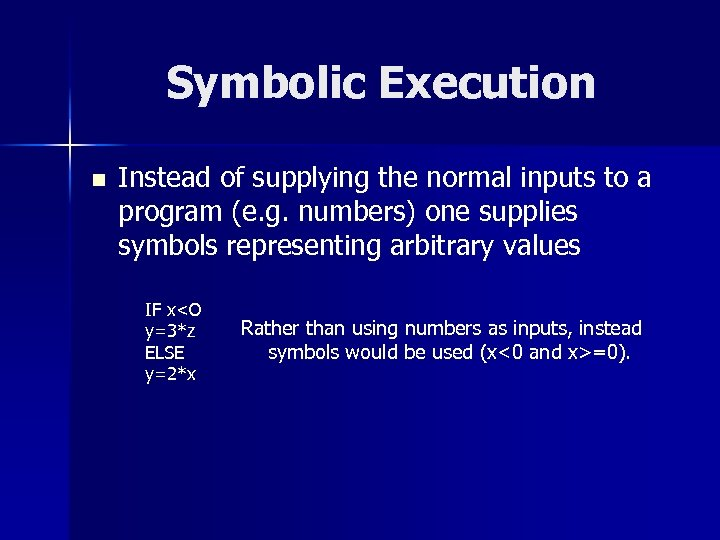Symbolic Execution n Instead of supplying the normal inputs to a program (e. g. numbers) one supplies symbols representing arbitrary values IF x=0).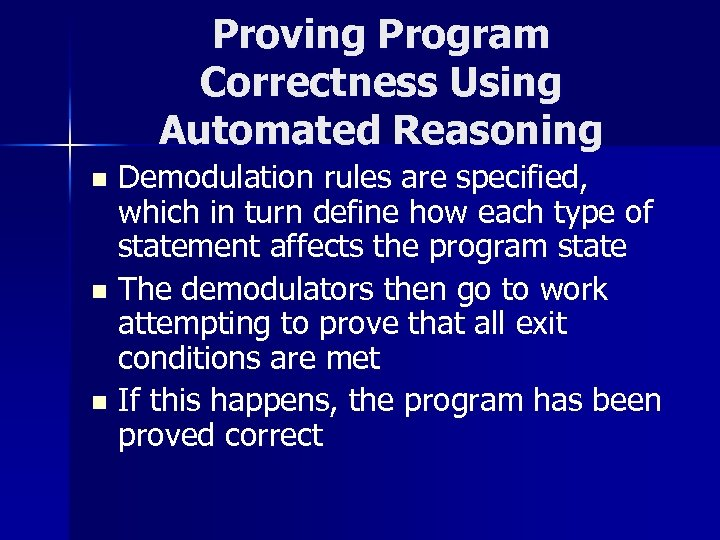Proving Program Correctness Using Automated Reasoning Demodulation rules are specified, which in turn define how each type of statement affects the program state n The demodulators then go to work attempting to prove that all exit conditions are met n If this happens, the program has been proved correct n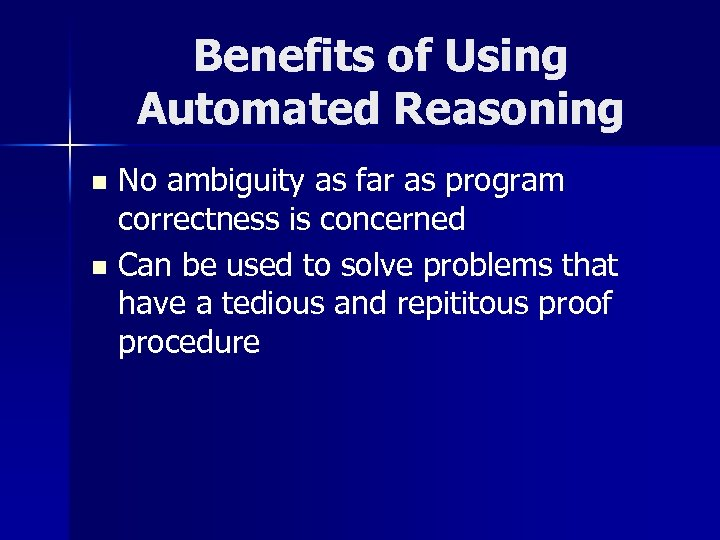Benefits of Using Automated Reasoning No ambiguity as far as program correctness is concerned n Can be used to solve problems that have a tedious and repititous proof procedure n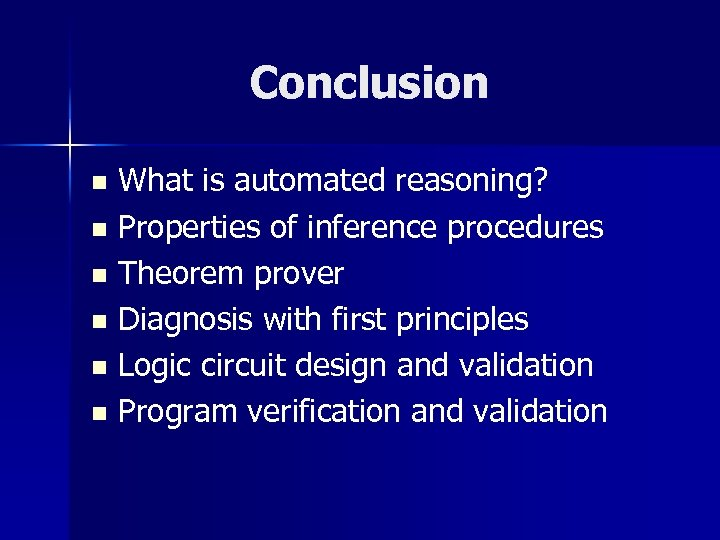Conclusion What is automated reasoning? n Properties of inference procedures n Theorem prover n Diagnosis with first principles n Logic circuit design and validation n Program verification and validation n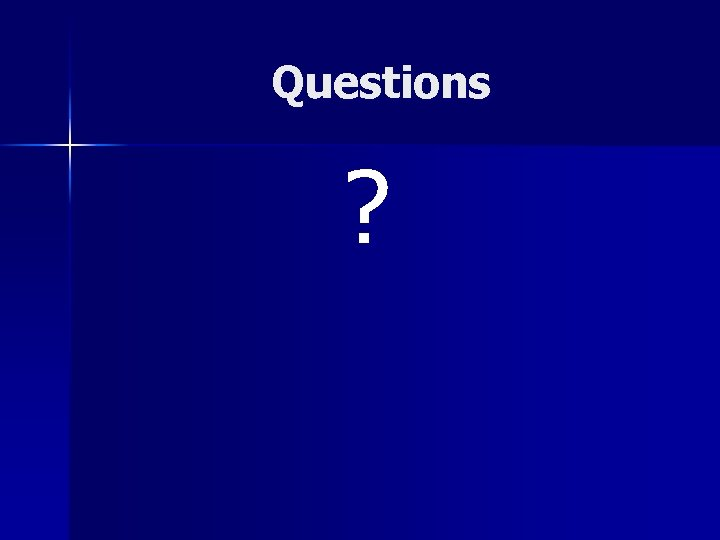Questions ?